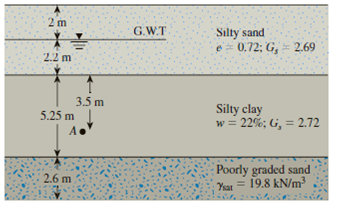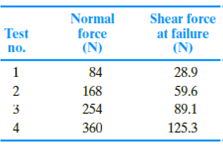Chapter 12, Problem 12.7PPrinciples of Geotechnical Enginee...

9th Edition
Braja M. Das + 1 other
ISBN: 9781305970939

Solutions

Chapter
SectionPrinciples of Geotechnical Enginee...

9th Edition
Braja M. Das + 1 other
ISBN: 9781305970939
Textbook Problem

Refer to Problem 12.6. In addition to the triaxial test, an unconfined compression test was conducted on an undisturbed sample of the same soil. If the compressive strength was found to be 95 kN/m2, determine the pore water pressure at failure for the unconfined specimen.12.6 Consider the silty clay soil in Problem 12.4. If a consolidated drained triaxial test is conducted on the same soil with a chamber-confining pressure of 105 kN/m2, what would be the deviator stress at failure?12.4 During a subsoil exploration program, undisturbed normally consolidated silty clay samples were collected in Shelby tubes from location A as shown in Figure 12.54.Figure 12.54Following are the results of four drained, direct shear tests conducted on the clay samples with each having a diameter of 63.5 mm and height of 32 mm.To determine

Find the pore water pressure at failure for the unconfined specimen.

Explanation

Given information:

The diameter (d) of clay sample is 63.5mm.

The height (h) of the clay sample is 32mm.

The compressive strength (σ1) is 95kN/m2.

Calculation:

Calculate the area of specimen (A) as follows:

A=πd24 (1)

Substitute 63.5mm for d in Equation (1).

A=π(63.5)24=12,661.264=3,165.32mm2(1m103mm)2=0.00316m2

Find the normal stress (σ) for Test No 1 using the formula as follows:

σ=NA (2)

Here, N is the normal force and A is the area of specimen.

Substitute 0.00316m2 for A and 84N for N in Equation (2).

σ=840.00316=26,582.27N/m2(1kN1,000N)=26.58kN/m2

Similarly calculate the normal stress (σ) for remaining Tests as shown in Table 1.

 Test no Normal force, N(N) Normal stress (σ′)(kN/m2) 1 84 26.58 2 168 53.16 3 254 80.38 4 360 113.92

Find the shear strength (τf) of the failure as follows:

τf=SA (3)

Here, S is shear force.

Substitute 28.9N for shear force and 0.00316m2 for A in Equation (3).

τf=28.90.00316=9,145.5N/m2(1kN1,000N)=9.14kN/m2

Find the angle of friction (ϕ) as shown below:

ϕ=tan1(τfσ) (4)

Here, σ is the normal stress.

Substitute 26.58kN/m2 for σ and 9.14kN/m2 for τf in Equation (4).

ϕ=tan1(9.1426.58)=18.976°

Similarly calculate the angle of friction (ϕ) and shear strength (τf) for remaining Tests as shown in Table 2.

 Test no Shear force, S (N) Shear strength, τf(kN/m2) Angle of friction (ϕ)(deg) 1 28.9 9.14 18.97 2 59.6 18.86 19.53 3 89.1 28.19 19.33 4 125.3 39.65 19.19

Find the drained angle of friction for the silty clay soil using the relation as follows:

Calculate the average value of angle of friction using Table 2.

ϕ=18.97+19.53+19.33+19.194=77

Still sussing out bartleby?

Check out a sample textbook solution.

See a sample solution

The Solution to Your Study Problems

Bartleby provides explanations to thousands of textbook problems written by our experts, many with advanced degrees!

Get Started

What is a hybrid firewall?

Principles of Information Security (MindTap Course List)

What is a slave motor?

Electric Motor Control

Replace the two forces shown by a force-couple system with the force acting at O.

International Edition---engineering Mechanics: Statics, 4th Edition

Describe the habits of successful interface designers.

Systems Analysis and Design (Shelly Cashman Series) (MindTap Course List)

What do the two pressure gauges on a regulator show?

Welding: Principles and Applications (MindTap Course List)